# Chapter 8: Intensity Models

“We must think about what our models mean, regardless of fit, or we will promulgate nonsense.”—Leland Wilkinson

packages: quantreg, xtable, ismev, gamlss, gamlss.cens
source code: source(“CountyWinds.R”)
third party: NONE

Interest is typically on the strongest. Hurricanes like Camille in 1969, Andrew in 1992, and Katrina in 2005 cause catastrophic damage.

It's important to have an estimate of when the next big one will occur. You also might want to know what influences the strongest hurricanes and if they are getting stronger.

Here we show you how to model hurricane intensity. The data are basin-wide lifetime highest intensities for individual tropical cyclones over the North Atlantic and county-level hurricane wind intervals.

We begin by considering trends using the method of quantile regression and then examine extreme-value models for estimating return period of the strongest hurricanes. We also look at modeling cyclone winds when the values are given by category and use Miami-Dade County as an example.

Here we consider cyclones above tropical storm intensity ($$\ge$$ 17 m s$$^{-1}$$) during the period 1967–2010, inclusive. The period is long enough to see changes but not too long that it includes intensity estimates before satellite observations.

We use 'intensity' and 'strength' interchangeably to mean the fastest wind inside the hurricane.

### Exploratory analysis

Consider the set of events defined by the location and wind speed at which a tropical cyclone first reaches its lifetime maximum intensity. The data are in the file LMI.txt.

Import and list the values in ten columns of the first six rows of the data frame by typing

con = "http://www.hurricaneclimate.com/storage/chapter-8/LMI.txt"

##         Sid   Yr Mo Da hr   lon WmaxS maguv
## 26637.5 941 1967  9  3 17 -52.2  70.5  27.5
## 26703.4 942 1967  9 20 10 -97.1 136.2   8.0
## 26747.2 943 1967  9 13  2 -51.0  94.5   4.2
## 26807.2 944 1967  9 13 20 -65.0  74.3   3.8
## 26849.5 945 1967  9 28 23 -56.9  47.3   9.0
## 26867   946 1967 10  3  0 -93.7  69.0   5.6


Interest centers on the smoothed intensity estimate at the time of lifetime maximum (WmaxS).

First convert the wind speeds from the operational units of knots to the SI units of m s$$^{-1}$$.

LMI.df$WmaxS = LMI.df$WmaxS * 0.5144


Next, determine the quartiles (0.25 and 0.75 quantiles) of the wind speed distribution. The quartiles divide the cumulative distribution function (CDF) into three equal-sized subsets.

quantile(LMI.df$WmaxS, c(0.25, 0.75))  ## 25% 75% ## 25.50 45.97  We find that 25% of the cyclones have a maximum wind speed less than 26 m s$$^{-1}$$ and 75% have a maximum wind speed less than 46 m s$$^{-1}$$ so that 50% of all cyclones have a maximum wind speed between 26 and 46 m s$$^{-1}$$ (interquartile range–IQR). Given a sample of maximum wind speeds $$w_1,\ldots, w_n$$, the $$\tau$$ th sample quantile is the $$\tau$$ th quantile of the corresponding empirical CDF. Formally, let $$W$$ be the highest storm intensity then the $$k$$ th $$q$$-quantile is defined as the value $$w$$ such that $p(W \le w) \ge \tau \hbox{ and } p(W \ge w) \ge 1 - \tau,$ where $$\tau=\frac{k}{n}$$. The CDF appears to have three distinct regions, indicated by the vertical lines. The function is nearly a straight line for intensities less than 40 m s$$^{-1}$$ and greater than 65 m s$$^{-1}$$. pt = seq(0, 1, 0.01) qp = quantile(LMI.df$WmaxS, prob = pt)
par(mfrow = c(1, 2), las = 1, mgp = c(2, 0.4, 0), tcl = -0.3)
plot(ecdf(LMI.df$WmaxS), xlab = "Wind speed [m/s]", ylab = "Cumulative distribution", main = "") abline(v = 40, col = "red") abline(v = 65, col = "red") rug(LMI.df$WmaxS)
mtext("a", side = 3, line = 1, adj = 0, cex = 1.1)
plot(pt, qp, type = "l", xlab = "Quantile", ylab = "Wind speed [m/s]", lwd = 2)
mtext("b", side = 3, line = 1, adj = 0, cex = 1.1)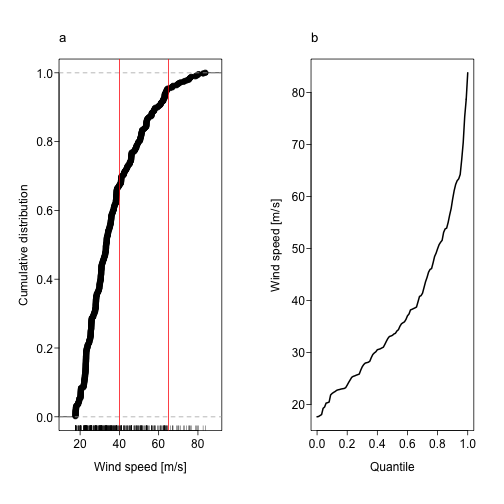Is there a trend in cyclone intensities? Start with a plot of your data. By specifying the first argument in the boxplot() function as a model formula you create a sequence of conditional box plots.

For example, to create a series of wind speed box plot conditional on year, type

boxplot(LMI.df$WmaxS ~ as.factor(LMI.df$SYear))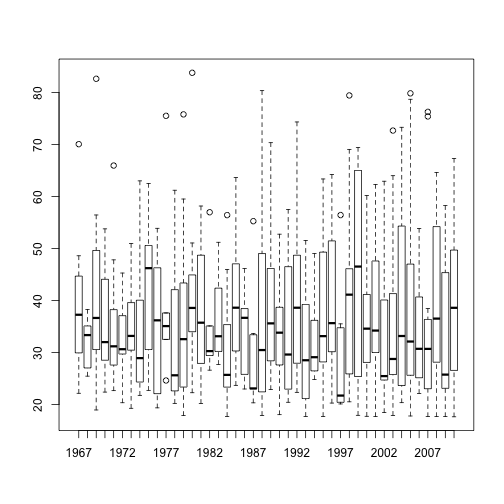The conditioning variable must be specified as a factor. The graph is useful for a quick look at the distribution of your wind speed data over time.

Earlier you created a series of box plots of the SOI by month that minimized the amount of redundant ink. Here you reuse this code, modifying it a bit, to create a series of wind speed box plots by year.

Begin by creating a vector of years and saving the length of the vector as a numeric object.

yrs = 1967:2010
n = length(yrs)


Next create the plot frame without the data and without the horizontal axis tic labels. You also add a label to the vertical axis. The fivenum() function lists the minimum, first quartile, median, third quartile, and maximum value in that order, so to obtain the median value from a vector of values called x, you type fivenum(x).

Thus you loop over each year indexed by i and plot the median wind speed value for that year as a point using the points() function. In the same loop you create vertical lines connecting the minimum with the first quartile and the third quartile with the maximum using the lines() function.

maxw = numeric()
plot(c(1967, 2010), c(15, 85), type = "n", xaxt = "n", bty = "n", xlab = "Year",
ylab = "Wind speed [m/s]")
axis(1, at = yrs, labels = yrs, cex = 0.5)
for (i in 1:n) {
fn = fivenum(LMI.df$WmaxS[LMI.df$SYear == yrs[i]])
points(yrs[i], fn, pch = 19)
lines(c(yrs[i], yrs[i]), c(fn, fn))
lines(c(yrs[i], yrs[i]), c(fn, fn))
maxw[i] = fn
}
abline(lm(maxw ~ yrs), col = "red", lwd = 2)
abline(lm(WmaxS ~ SYear, data = LMI.df), lwd = 2)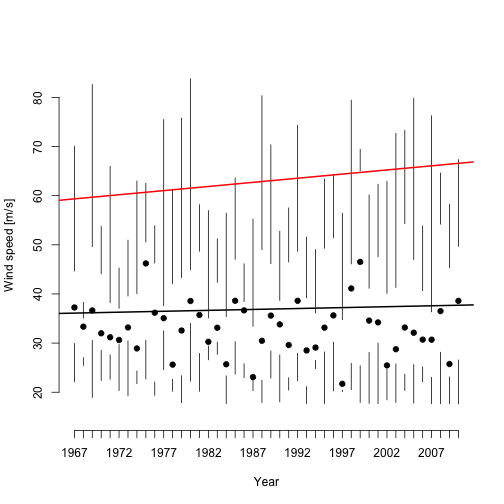Here we added the least-squares regression line about the annual mean lifetime highest wind speed (black line) and the least-squares regression line about the annual lifetime highest wind speed (red).

While there is no upward or downward trend in the average cyclone intensity, there is an upward trend to the set of strongest cyclones.

The theory of maximum potential intensity, which relates intensity to ocean heat, refers to a theoretical limit given the thermodynamic conditions (Emanuel 1988). So the upward trend in the observed lifetime maximum intensity is physically consistent with what you expect given the increasing ocean temperature.

It is informative then to explore the relationship of lifetime highest wind speed to ocean temperature. Get the monthly North Atlantic sea-surface temperature (SST) data by typing

con = "http://www.hurricaneclimate.com/storage/chapter-8/SST.txt"


Here you subset the data on years since 1967, and keep June values only.

lg = SST$Year >= 1967 sst.df = data.frame(Yr = SST$Year[lg], sst = SST$Jun[lg])  Next merge your SST data frame with your cyclone intensity data. This is done using the merge() function. Merge is performed on the common column name Yr as specified with the by argument. lmisst.df = merge(LMI.df, sst.df, by = "Yr") head(lmisst.df[c("Yr", "WmaxS", "sst")])  ## Yr WmaxS sst ## 1 1967 36.27 21 ## 2 1967 70.06 21 ## 3 1967 48.59 21 ## 4 1967 38.23 21 ## 5 1967 24.33 21 ## 6 1967 35.52 21  There are more instances of Yr in the intensity data frame (one for each cyclone) so the June SST values in the SST data frame get duplicated. All cyclones for a particular year get the same SST value as it should be. You are interested in regressing cyclone intensity on SST as you did above on the year, but the SST values are continuous rather than discrete. So you first create SST intervals. This is done with the cut() function. brk = quantile(lmisst.df$sst, prob = seq(0, 1, 0.2))
sst.i = cut(lmisst.df$sst, brk, include.lowest = TRUE)  Your cuts divide the SST values into five equal quantiles (pentiles). The intervals represent categories of much below normal, below normal, normal, above normal, and much above normal SST. The choice of quantiles is a compromise between having enough years for a given range of SSTs and having enough quantiles to assess differences. Repeat this procedure for your SOI data. You create a merged data frame and cut the SOI values into pentads. con = "http://www.hurricaneclimate.com/storage/chapter-8/SOI.txt" SOI = read.table(con, header = TRUE) lg = SOI$Year >= 1967
soi.df = data.frame(Yr = SOI$Year[lg], soi = SOI$Sep[lg])
lmisoi.df = merge(LMI.df, soi.df, by = "Yr")
brk = quantile(lmisoi.df$soi, prob = seq(0, 1, 0.2)) soi.i = cut(lmisoi.df$soi, brk, include.lowest = TRUE)


Finally, you create a series of box plots corresponding to the SST intervals. Begin by creating a character vector of horizontal axis labels corresponding to the SST intervals and, to simplify the code, save the wind speeds as a vector.

xlabs = c("MB", "B", "N", "A", "MA")
W = lmisst.df$WmaxS y = boxplot(W ~ sst.i, plot = FALSE)  Plot and add regression lines through the medians and third quartile values using the saved statistics of the box plot and regressing on the sequence from one to five. Repeat the code using the September SOI covariate and create two box plots. par(mfrow = c(1, 2), las = 1, mgp = c(2, 0.4, 0), tcl = -0.3) y = boxplot(W ~ sst.i, plot = FALSE) boxplot(W ~ sst.i, notch = TRUE, names = xlabs, xlab = "June SST pentile", ylab = "Wind speed [m/s]", cex.axis = 0.7) x = 1:5 abline(lm(y$stats[3, ] ~ x), lwd = 2)
abline(lm(y$stats[4, ] ~ x), col = "red", lwd = 2) mtext("a", side = 3, line = 1, at = 0, cex = 1.1) y = boxplot(W ~ soi.i, plot = FALSE) boxplot(W ~ soi.i, notch = TRUE, names = xlabs, xlab = "September SOI pentile", ylab = "Wind speed [m/s]", cex.axis = 0.7) abline(lm(y$stats[3, ] ~ x), lwd = 2)
abline(lm(y$stats[4, ] ~ x), col = "red", lwd = 2) mtext("b", side = 3, line = 1, at = 0, cex = 1.1)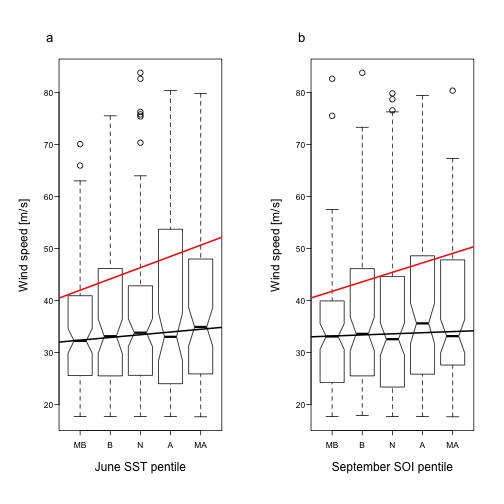The first pentad is the lowest 20% of all values. The upper and lower limits of the boxes represent the first and third quartiles of cyclone intensity. The median for each group is represented by the horizontal bar in the middle of each box. Notches on the box sides represent an estimated confidence interval about the median. The full range of the observed intensities in each group is represented by the horizontal bars at the end of the dashed whiskers. In cases where the whiskers would extend more than one and half times the interquartile range, they are truncated and the remaining outlying points are indicated by open circles. The red line is the best-fit line through the upper quartile and the black line is through the medians. The box plot summarizes the distribution of maximum storm intensity by pentiles of the covariate. The graphs show a tendency for the upper quantiles of cyclone intensity values to increase with both SST and SOI. As SST increases so does the intensity of the strongest cyclones. Also as SOI increases (toward more La Nina-like conditions) so does the intensity of the cyclones. Results from this exploratory analysis give you reason to continue your investigation. The next step is to model these data. The box plots provide evidence that a model for the mean will not capture the relationships as the trends are larger for higher quantiles. So instead of linear regression you use quantile regression. ### Quantile regression The quantile function and the conditional box plots shown above are useful for exploratory analysis. They are adequate for describing and comparing univariate distributions. However, since you are interested in modeling the relationship between a response variable (intensity) and the covariates (SST and SOI) it is necessary to introduce a regression-type model for the quantile function. Quantile regression extends ordinary least squares regression model to conditional quantiles of the response variable. Although you used linear regression on the conditional quantiles in the plots above, this is not the same as quantile regression on the covariates. Quantile regression allows you to examine the relationship without the need to consider discrete levels of the covariate. Ordinary regression model specifies how the mean changes with changes in the covariates while the quantile regression model specifies how the quantile changes with changes in the covariates. Quantile regression relies on empirical quantiles, but uses parameters to assess the relationship between the quantile and the covariates. The quantile regression model with two covariates is given by $\hat \mu(\tau) = \hat \beta_0(\tau) + \hat \beta_1(\tau) x_1 + \hat \beta_2 (\tau) x_2\\$ where $$\hat \mu(\tau)$$ is the predicted conditional quantile of tropical cyclone intensity ($$W$$) and where the $$\hat \beta_i$$'s are obtained by minimizing the piecewise linear least absolute deviation function given by $\frac{1-\tau}{n}\sum_{w_i < q_i}|w_i - q_i| + \frac{\tau}{n}\sum_{w_i > q_i}|w_i - q_i|.$ for a given $$\tau$$, where $$q_i$$ is the predicted $$\tau$$ quantile corresponding to observation $$i$$ ($$\hat \mu_i(\tau)$$). The value of a simple trend analysis (involving only one variable—usually time) is limited by the fact that other explanatory variables also might be trending. It is well known that the ENSO cycle can significantly alter the frequency and intensity of hurricane activity on the seasonal time scale. A trend over time in hurricane intensity could simply reflect a change in this cycle. Thus it is important to look at the trend after controlling for this important factor. Here we show the trend as a function of Atlantic SST after controlling for the ENSO cycle. Thus we answer the question of whether the data support the contention that the increasing trend in the intensity of the strongest hurricanes is related to an increase in ocean warmth conditional on ENSO. Here we use the quantreg package for performing quantile regression developed by Roger Koenker. Load the package and print a BibTeX citation. require(quantreg) x = citation(package = "quantreg") toBibtex(x)  ## @Manual{, ## title = {quantreg: Quantile Regression}, ## author = {Roger Koenker}, ## year = {2012}, ## note = {R package version 4.94}, ## url = {http://CRAN.R-project.org/package=quantreg}, ## }  Begin with median regression. Here $$\tau$$ is set to 0.5 and is specified with the tau argument. The function rq performs the regression. The output is assigned to the object qrm. Year = lmisst.df$Yr
W = lmisst.df$WmaxS SOI = lmisoi.df$soi
SST = lmisst.df$sst qrm = rq(W ~ Year + SST + SOI, tau = 0.5)  By default a simplex method is used to fit the regression. It is a variant of the Barrodale and Roberts (1974) approach described in Koenker and d'Orey (1987). You obtain a concise summary of the regression results by typing qrm  ## Call: ## rq(formula = W ~ Year + SST + SOI, tau = 0.5) ## ## Coefficients: ## (Intercept) Year SST SOI ## 238.0389 -0.2210 11.0087 0.8268 ## ## Degrees of freedom: 500 total; 496 residual  The output shows the estimated coefficients and information about the degrees of freedom. You find that the median lifetime intensity decreases with year (negative trend) and increases with both SST and the SOI. More details are available through the the summary() method. summary(qrm)  ## ## Call: rq(formula = W ~ Year + SST + SOI, tau = 0.5) ## ## tau:  0.5 ## ## Coefficients: ## coefficients lower bd upper bd ## (Intercept) 238.03885 11.67072 414.77836 ## Year -0.22095 -0.33418 -0.05102 ## SST 11.00873 0.73362 16.98951 ## SOI 0.82685 -0.65041 1.92524  The table gives the estimated coefficients and confidence intervals (95%) for these parameters. The confidence intervals are computed by the rank inversion method developed in Koenker (2005). The confidence interval includes zero for the SOI indicating this term is not significant in explaining the median per cyclone intensity. However, the SST variable is significant and positive. The relationship indicates that for every 1$\circ$$C increase in SST the median intensity increases by 11 m s$${-1}$. But this seems too large (by an order of magnitude) given the box plot above and the range of SST values. range(SST)  ##  20.78 21.83  The problem stems from the other variables in the model. To see this, refit the regression model removing the variables that are not significant. qrm2 = rq(W ~ SST, tau = 0.5) summary(qrm2)  ## Warning: Solution may be nonunique  ## ## Call: rq(formula = W ~ SST, tau = 0.5) ## ## tau:  0.5 ## ## Coefficients: ## coefficients lower bd upper bd ## (Intercept) -14.227 -141.106 63.122 ## SST 2.226 -1.438 8.176  Now the relationship indicates that for every 1 K increase in SST the median intensity increases by 2.23 m s$$^{-1}$$. This amount is not statistically significant as you might have guessed from your exploratory plot. The theory of maximum potential intensity relates a theoretical highest wind speed to ocean temperature so it is interesting to consider quantiles above the median. You repeat the modeling exercise using $$\tau$$ = 0.9. Here again you find year and SOI not significant so you exclude them in your final model. summary(rq(W ~ SST, tau = 0.9), se = "iid")  ## ## Call: rq(formula = W ~ SST, tau = 0.9) ## ## tau:  0.9 ## ## Coefficients: ## Value Std. Error t value Pr(>|t|) ## (Intercept) -307.30045 68.69143 -4.47364 0.00001 ## SST 17.15850 3.21853 5.33117 0.00000  Here instead of the rank-inversion CI you obtain a table of coefficients that includes standard errors, $$t$$-statistics, and $$p$$-values using the se=“iid” argument from the summary() function. As anticipated from theory and your exploratory data analysis, you see a statistically significant positive relationship between cyclone intensity and SST for the top 10% of the strongest tropical cyclones. The estimated coefficient indicates that for every 1 K increase in SST the upper percentile intensity increases by -0.2 m s$$^{-1}$$. Other options exist for computing standard errors including a bootstrap approach (se=“boot”; see Koenker 2005). iid = summary(rq(W ~ SST, tau = 0.9), se = "iid") boot = summary(rq(W ~ SST, tau = 0.9), se = "boot") percinc = (boot$coef[2, 2] - iid$coef[2, 2])/iid$coef[2, 2] * 100


The bootstrap standard error is 3.69 (difference of 15%). The larger standard error results in a significance level that is somewhat less, but the results still provide conclusive evidence of a climate change signal.

To visualize the intensity-SST relationship in more detail you plot several quantile regression lines on a scatter plot. For reference you include the least-squares regression line.

The code is given below. Note that you use type=“n” in the plot function and use the points() function to add the points last so the lines do not cover them.

par(las = 1, mgp = c(2, 0.4, 0), tcl = -0.3)
plot(SST, W, type = "n", xlab = "SST [C]", ylab = "Wind speed [m/s]")
abline(lm(W ~ SST), col = "red", lwd = 2)
taus = c(0.1, 0.25, 0.5, 0.75, 0.9)
for (i in 1:length(taus)) {
abline(rq(W ~ SST, tau = taus[i]), col = "gray", lwd = 2)
}
points(SST, W, pch = 19, cex = 0.4)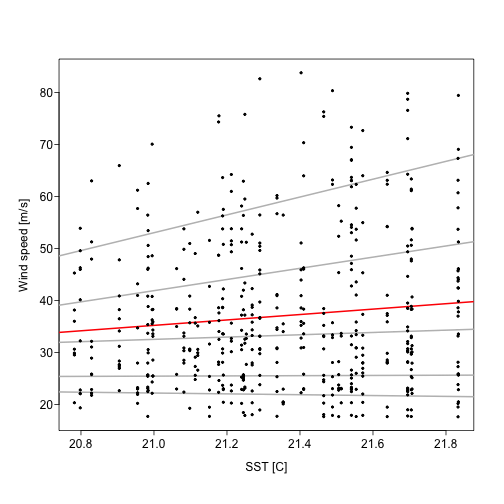The 0.1, 0.25, 0.5, 0.75, and 0.9 quantile regression lines are shown in gray and the least squares regression line about the mean is shown in red. Trend lines are close to horizontal for the weaker tropical cyclones but have a significant upward slope for the stronger cyclones.

To see all the distinct quantile regressions for a particular model you specify a tau=-1. For example, save the quantile regressions of wind speed on SST and SOI in an object by typing

model = rq(W ~ SST + SOI, tau = -1)


This will cause the rq() function to find the entire sample path of the quantile process. The returned object is of class rq.process.

You plot the regression coefficients for each variable in the model as a function of quantile by typing

plot.rq.process(model)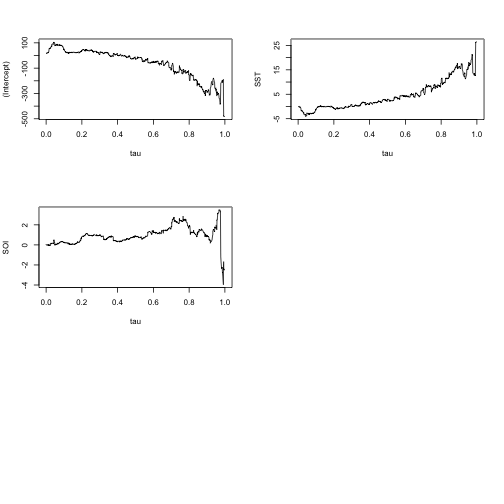Another plot at discrete quantiles is made by typing.

model = rq(W ~ SST + SOI, tau = seq(0.025, 0.975, 0.05))
labs = round(quantile(W, seq(0.2, 0.8, 0.2)))
par(las = 1, mgp = c(2, 0.4, 0), tcl = -0.3)
plot(summary(model, se = "boot"), parm = 2, lcol = "transparent", xaxt = "n",
mar = c(5, 5, 4, 2) + 0.1, pch = 16, lwd = 2, ylab = expression(paste(beta[SST],
" [m/s/C]")), xlab = expression(paste("Wind speed quantile [", tau,
"]")), main = "")
grid()
abline(h = 0)
axis(3, at = seq(0.2, 0.8, 0.2), labels = labs)
mtext("Lifetime highest wind speed [m/s]", side = 3, line = 2)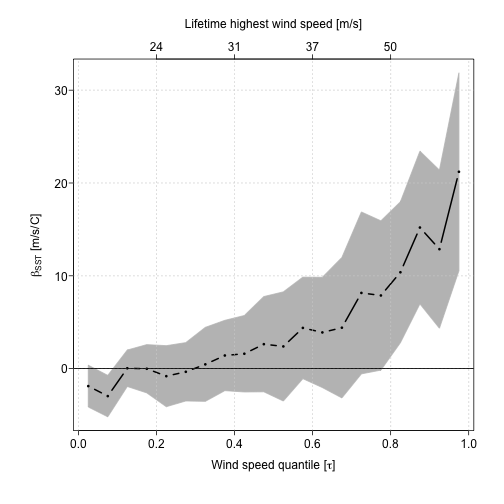Values of $$\tau$$ range from 0.025 to 0.975 in intervals of 0.05. The 95% confidence band (gray) is based on a bootstrap method. The plot shows the rising trend of the most intense hurricanes as the ocean temperatures rise after accounting for the El Nino.

The trends depart from zero for quantiles above about 0.4 and become significant for cyclones exceed about 50 m s$$^{-1}$$. Additional capabilities for quantile modeling and inference are available in the quantreg package. Next we consider a model for the most intense hurricanes.

## Fastest Winds

Eighty percent of all hurricane damage is caused by fewer than 20% of the worst events (Jagger et al. 2008). The rarity of severe hurricanes implies that empirical models that estimate the probability of the next big one will be unreliable.

Extreme value theory provides a framework for statistically modeling these rare wind events. Here you employ these models on hurricane wind speeds. This is particularly important since you saw in the previous section that the strongest are getting stronger.

### Exploratory analysis

Here you plot the lifetime maximum wind speeds for all North Atlantic tropical cyclones from the period 1967–2010 as a histogram. You use the same dataset as before.

W = LMI.df$WmaxS hist(W, main = "", las = 1, col = "gray", border = "white", xlab = "Wind Speed (m/s)") rug(W)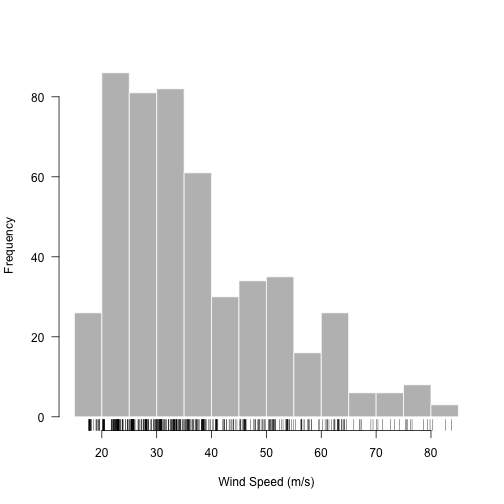The default bin width is 5 m s$$^{-1}$$ although the minimum intensity is 17.6 m s$$^{-1}$$. The distribution peaks between 20 and 40 m s$$^{-1}$$ and has a long right tail. For a model of the fastest winds you want to include enough of these high values that your parameter estimates are reliable (they don't change by much if you add or remove a few values). But you also want to be careful not to include too many to ensure that you exclude values representing the more typical cyclones. ### Return period The return period is the average recurrence interval, where the recurrence interval is the time between successive cyclones of a given intensity or stronger (events). Suppose you define an event as a hurricane with a threshold intensity of 75 m s$$^{-1}$$, then the annual return period is the inverse of the probability that such an event will be exceeded in any one year. For instance, a 10-year hurricane event has a 1/10 = 0.1 or 10% chance of having an intensity exceeding a threshold level in any one year and a 50-year hurricane event has a 0.02 or 2% chance of having an intensity exceeding a higher threshold level in any one year. These are statistical statements. On average, a 10-year event will occur once every 10 years. The interpretation requires that for a year or set of years in which the event does not occur, the expected time until it occurs next remains 10 years, with the 10-year return period resetting each year. There is a monotonic relationship between the intensity of the hurricane event (return level) and the return period. The return period for a 75 m s$$^{-1}$$ return level must be longer than the return period for a 70 m s$$^{-1}$$ return level. The empirical relationship is expressed as $\hbox{RP}=\frac{n+1}{m}$ where $$n$$ is the number of years in the record and $$m$$ is the intensity rank of the event. Sometimes expressed as $$n/(m-0.5)$$ instead. You use this formula to estimate return periods for your set of hurricanes. First assign the record length and sort the lifetime maximum wind speeds in decreasing order. Then list the speeds of the six most intense hurricanes. n = length(1967:2010) Ws = sort(W, decreasing = TRUE) round(Ws, 1)[1:6]  ##  83.8 82.6 80.3 79.8 79.4 78.7  Finally, compute the return period for these six events using the above formula rounding to the nearest year. m = rev(rank(Ws)) round((n + 1)/m, 0)[1:6]  ##  45 22 15 11 9 8  Thus a 83.8 m s$$^{-1}$$ hurricane has a return period of 45 years and a 78.7 m s$$^{-1}$$ hurricane has a return period of 8 years. Said another way, you can expect a hurricane of at least 80.3 m s$$^{-1}$$ once every 15 years. The threshold wind speed for a given return period is called the return level. Your goal is a statistical model that provides a continuous estimate of the return level (threshold intensity) for a set of return periods. A model is more useful than a set of empirical estimates because it provides a smoothed return level estimate for all return periods and it allows you to estimate the return level for a return period longer than your data record. Rupp and Lander (1996) use the method of moments on annual peak winds over Guam to determine the parameters of an extreme value distribution leading to estimates of return periods for extreme typhoon winds. Heckert et al. (1998) use the peaks-over-threshold method and a reverse Weibull distribution to obtain return periods for extreme wind speeds at locations along the U.S. coastline. Walshaw (2000) use a Bayesian approach to model extreme winds jointly from tropical and non-tropical systems. The approach allows them to take advantage of information from nearby sites Jagger and Elsner (2006) use a maximum-likelihood and Bayesian approach to model tropical cyclone winds in the vicinity of the United States conditional on climate factors. The approach allows them to take advantage of older, less reliable, data. Here you use functions in the ismev package Coles and Stephenson (2011) to fit an extreme value model for hurricane winds using the method of maximum likelihoods. We begin with some background material. An excellent introduction is provided in Coles (2001). ### Extreme value theory Extreme value theory is a branch of statistics. It concerns techniques and models for describing the rare event rather than the typical, or average, event. It is similar to the central limit theory. Both consider the limiting distributions of independent identically distributed (iid) random variables under an affine transformation (Linear transformation followed by a translation). According to the central limit theorem, the mean value of an iid random variable $$x$$ converges to a normal distribution with mean 0 and variance 1 under the transformation $$(\bar x - \mu)/\sqrt{n\sigma^2})$$, where $$\mu$$ and $$\sigma$$ are the mean and standard deviation of $$x$$, respectively. Similarly, if the distribution of the maxima converges, then it must converge to a member of the generalized extreme value (GEV) family (Embrechts et al. 1997). The maxima of most continuous random variables converge to a random variable. This asymptotic argument is used to motivate the use of extreme value models in the absence of empirical or physical evidence for assigning an extreme level to a process. However, the argument does not hold for the maxima of discrete random variables including the Poisson and negative binomial. Although by definition extreme values are scarce, an extreme value model allows you to estimate return periods for hurricanes that are stronger than the strongest one in your data set. In fact, your goal is to quantify the statistical behavior of hurricane activity extrapolated to unusually high levels. Extreme value theory provides models for extrapolation. Given a set of observations from a continuous process, if you generate a sample from the set, take the maximum value from the sample, and repeat the procedure many times, you obtain a distribution that is different from that of the original (parent) distribution. For instance, if the original distribution is described by a normal, the distribution of the maxima is quantified by a Gumbel distribution. To see this plot a density curve for the standard normal distribution and compare it to the density curve of the maxima from samples of size 100 taken from the same distribution. Here you generate 1000 samples saving the maxima in the vector m. par(mfrow = c(1, 2), las = 1, mgp = c(2, 0.4, 0), tcl = -0.3) curve(dnorm(x), from = -4, to = 4, ylab = "Density", lwd = 2) mtext("a", side = 3, line = 1, adj = 0, cex = 1.1) m = numeric() for (i in 1:1000) m[i] = max(rnorm(100)) plot(density(m), xlab = "Maxima of x", main = "", lwd = 2) mtext("b", side = 3, line = 1, adj = 0, cex = 1.1)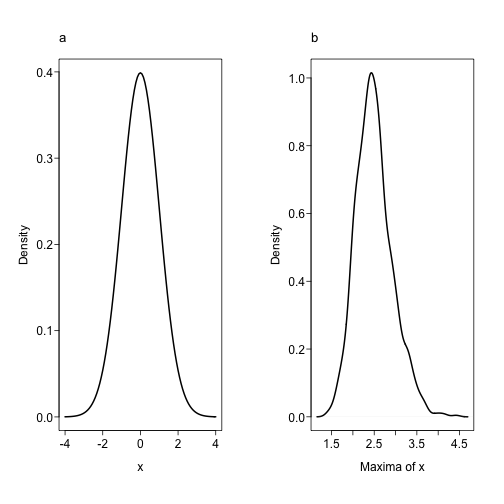The maxima are described by a GEV distribution that is shifted relative to the parent distribution and positively skewed. The three parameters of the GEV distributions are determined by the values in the tail portion of the parent distribution. ### Generalized Pareto distribution A GEV distribution fits the set of values consisting of the single strongest hurricane each year. Alternatively, consider the set of per-cyclone lifetime strongest winds in which you keep all values exceeding a given threshold level, say 60 m s$$^{-1}$$. Some years will contribute no values to your set and some years will contribute two or more values. A two-parameter generalized Pareto distribution (GPD) family describes this set of fast winds. The threshold choice is a compromise between retaining enough tropical cyclones to estimate the distribution parameters with sufficient precision, but not too many that the intensities fail to be described by a GPD. Specifically, given a threshold wind speed $$u$$ you model the exceedances, $$W-u$$, as samples from a GPD family so that for an individual hurricane with maximum winds $$W$$, the probability that $$W$$ exceeds any value $$v$$ given that it is above the threshold $$u$$ is given by $p(W > v | W > u) = \left\{\begin{array}{cc} \exp([-(v-u)]/\sigma) & \mbox{ when } \xi = 0 \\ ( 1+\frac{\xi}{\sigma} [v-u])^{-1/\xi} & \mbox{otherwise} \\ \end{array} \right.$ where $$\sigma > 0$$ and $$\sigma + \xi (v-u) \ge 0$$. The parameters $$\sigma$$ and $$\xi$$ are scale and shape parameters of the GPD, respectively. Thus you can write $$p(W > v | W > u)$$ = GPD$$(v-u |,\sigma, \xi)$$. To illustrate, copy the following code to create a function sGpd() for the exceedance probability of a GPD. sGpd = function(w, u, sigma, xi) { d = (w - u) * (w > u) sapply(xi, function(xi) if (xi == 0) exp(-d/sigma) else ifelse(1 + xi/sigma * d < 0, 0, (1 + xi/sigma * d)^(-1/xi))) }  Given a threshold intensity $$u$$, the function computes the probability that a hurricane at this intensity or higher picked at random will have a maximum wind speed of at least $$W$$. The probability depends on the scale and shape parameters. For instance, given a scale of 10 and a shape of 0, the probability that a random hurricane will have a maximum wind speed of at least 70 m s$$^{-1}$$ is obtained by typing sGpd(w = 70, u = 60, sigma = 10, xi = 0)  ##  0.3679  The scale parameter controls how fast the probability decays for values near the threshold. The decay is faster for smaller values of $$\sigma$$. The shape parameter controls the length of the tail. For negative values of $$\xi$$ the probability is zero beyond a certain intensity. With $$\xi$$ = 0 the decay is exponential. For positive values of $$\xi$$ the tail is described as 'heavy' or 'fat' indicating a decay in the probabilities gentler than logarithmic. The figure below illustrates exceedance curves for different values of $$\sigma$$ with $$\xi=0$$ and for different values of $$\xi$$ with $$\sigma=10$$ keeping the threshold value at 60~m s$$^{-1}$$. par(mfrow = c(1, 2), las = 1, mgp = c(2, 0.4, 0), tcl = -0.3) curve(sGpd(x, u = 60, sigma = 5, xi = 0), from = 60, to = 100, lwd = 2, col = "green", xlab = "Wind speed [m/s]", ylab = "Exceedance probability") curve(sGpd(x, u = 60, sigma = 10, xi = 0), from = 60, to = 100, add = TRUE, lwd = 2) curve(sGpd(x, u = 60, sigma = 15, xi = 0), from = 60, to = 100, add = TRUE, lwd = 2, col = "red") legend("topright", legend = c("sigma = 5", "sigma = 10", "sigma = 15"), lwd = 2, col = c(3, 1, 2), cex = 0.8) mtext("a", side = 3, line = 1, adj = 0, cex = 1.1) curve(sGpd(x, u = 60, sigma = 10, xi = -0.5), from = 60, to = 100, lwd = 2, col = "green", xlab = "Wind speed [m/s]", ylab = "Exceedance probability") curve(sGpd(x, u = 60, sigma = 10, xi = 0), from = 60, to = 100, add = TRUE, lwd = 2) curve(sGpd(x, u = 60, sigma = 10, xi = 0.5), from = 60, to = 100, add = TRUE, lwd = 2, col = "red") legend("topright", legend = c("xi = -0.5", "xi = 0", "xi = +0.5"), lwd = 2, col = c(3, 1, 2), cex = 0.8) mtext("b", side = 3, line = 1, adj = 0, cex = 1.1)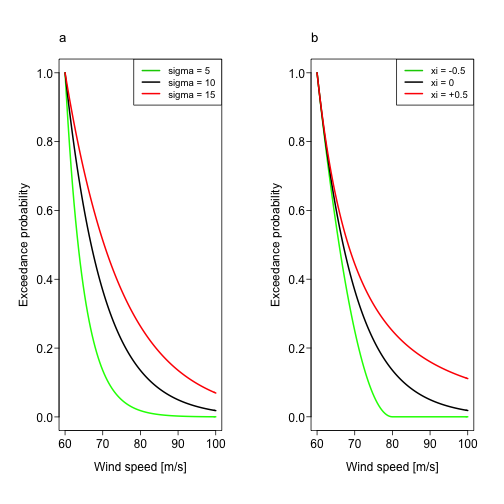### Extreme intensity model Given your set of lifetime maximum wind speeds (W), you use the gpd.fit() function from the ismev package (Coles, 2001) to find the scale and shape parameters of the GPD using the method of maximum likelihood. Here you set the threshold wind speed to 62 m s$$^{-1}$$ as a compromise between high enough to capture only the strongest cyclones but low enough to have a sufficient number of wind speeds. The output is saved as a list object and printed to your screen. require(ismev) con = "http://www.hurricaneclimate.com/storage/chapter-8/LMI.txt" LMI.df = read.table(con, header = TRUE) W = LMI.df$WmaxS * 0.5144
model = gpd.fit(W, threshold = 62)

## $threshold ##  62 ## ##$nexc
##  42
##
## $conv ##  0 ## ##$nllh
##  124
##
## $mle ##  9.8343 -0.3342 ## ##$rate
##  0.084
##
## $se ##  2.7960 0.2443  This is a probability model that specifies the chance of a random hurricane obtaining any intensity value given that it has already reached the threshold. The function prints the threshold value, the number of extreme winds in the data set (nexc) as defined by the threshold, the negative log-likelihood value (nllh), the maximum likelihood parameter estimates (mle) and the rate, which is the number of extreme winds divided by the total number of cyclones (per cyclone rate). You use your sGpd() function to compute probabilities for a sequence of winds from the threshold value to 85 m s$$^{-1}$$ in increments of 0.1 m s$$^{-1}$$. v = seq(63, 85, 0.1) p = sGpd(v, u = 62, sigma = model$mle, xi = model$mle)  You then use the plot method to graph them. plot(v, p, type = "l", lwd = 2, xlab = "Wind Speed (m/s)", ylab = "p(W > v | W > 62)")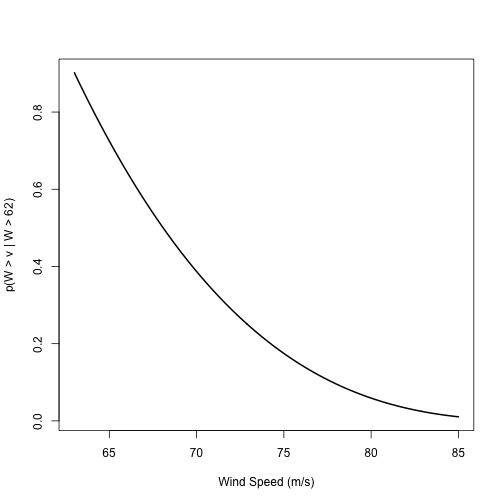To turn the per cyclone rate into an annual rate you divide the number of extreme winds by the record length. rate = model$nexc/length(1967:2010)
rate

##  0.9545


Thus the annual rate of hurricanes at this intensity or higher over the 44 years in the data set is 0.95 cyclones per year.

Recall from the Poisson distribution that this implies a

round((1 - ppois(0, rate)) * 100, 2)

##  61.5


percent chance that next year a hurricane will exceed this the threshold.

### Intensity and frequency model

The GPD describes hurricane intensities above a threshold wind speed. The Poisson distribution describes hurricane frequency.

Let the annual number of hurricanes whose lifetime maximum intensity exceeds $$u$$ have a Poisson distribution with mean rate $$\lambda_u$$. Then the average number of hurricanes with winds exceeding $$v$$ (where $$v \ge u$$) is given by $\lambda_v = \lambda_u \times p(W > v | W > u)$ This allows you to model hurricane occurrence separate from hurricane intensification. This is helpful because processes that govern hurricane formation are not necessarily the same as the processes that govern intensification.

From a practical perspective, rather than a return rate per hurricane occurrence, the above specification allows you to obtain an annual return rate on the extreme winds. This is more meaningful for the business of insurance.

Now, the probability that the highest lifetime maximum intensity in a given year will be less than $$v$$ is $p(W_{\hbox{max}} \le v) = \exp(-\lambda_v) \\ = \exp[-\lambda_u \times \hbox{GPD}(v-u | \sigma, \xi)]$ The return period RP is the inverse of the probability that $$W_{\hbox{max}}$$ exceeds $$v$$, where $$v$$ is called the return level. You compute the return period and create a return period plot using

rp = 1/(1 - exp(-rate * p))
plot(rp, v, type = "l", lwd = 2, log = "x", xlab = "Return Period (yr)", ylab = "Return Level (m/s)")
grid(equilogs = FALSE)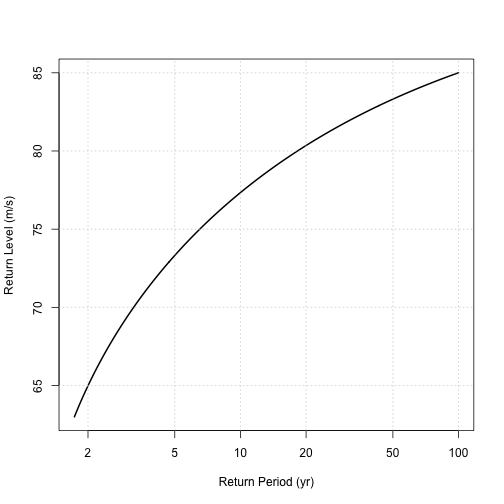Return levels increase with increasing return period. The model estimates an 81 m s$$^{-1}$$ hurricane will occur on average once every 27 years and an 85 m s$$^{-1}$$ hurricane will occur on average once every 100 years.

Based on the results from the quantile regression, these return periods might be getting shorter.

### Confidence intervals

v = 73
p = sGpd(v, u = 62, sigma = model$mle, xi = model$mle)
rp = round(1/(1 - exp(-rate * p)))


You obtain confidence limits on the return period estimates using a bootstrap approach.

Suppose you want a 95% CI on the return period of a 73 m s$$^{-1}$$ hurricane. Your model tells you that the best estimate for the return period is 5 years.

To obtain the CI you randomly sample your set of wind speeds with replacement to create a bootstrap replicate. You run your model on this replicate and get an estimate of the return period. You repeat this procedure 1000 times each time generating a new return period estimate.

You then treat the bootstrapped return periods as a distribution and find the lower and upper quantiles corresponding to the 0.025 and 0.975 probabilities.

To implement this procedure you type

thr = 62
v = 73
rps = numeric()
m = 1000
for (i in 1:m) {
Wbs = sample(W, size = length(W), replace = TRUE)
modelbs = gpd.fit(Wbs, threshold = thr, show = FALSE)
ps = sGpd(v, u = thr, sigma = modelbs$mle, xi = modelbs$mle)
rps[i] = 1/(1 - exp(-rate * ps))
}

## Warning: NaNs produced

## Warning: NaNs produced

ci = round(quantile(rps, probs = c(0.025, 0.975)))


The procedure provides a 95% CI of (3, 9) years about the estimated 5-year return period for a 73 m s$$^{-1}$$ hurricane. You can estimate other CIs (e.g., 90%) by specifying different percentiles in the quantile() function.

### Threshold intensity

The GPD model requires a threshold intensity $$u$$. The choice is a tradeoff between an intensity high enough that the positive residual values ($$W-u \ge 0$$) follow a GPD, but low enough that there are values to accurately estimate the GPD parameters.

For an arbitrary intensity level you can compute the average of the positive residuals (excesses). For example, at an intensity of 60~m s$$^{-1}$$, the mean excess in units of m s$$^{-1}$$ is

mean(W[W >= 60] - 60)

##  7.995


By increasing the level, say to 70~m s$$^{-1}$$, the mean excess decreases to 6.23 m s$$^{-1}$$.

In this way you compute a vector of mean excesses for a range of potential threshold intensities. The relationship between the mean excess and threshold is linear if the residuals follow a GPD.

A plot of the mean excess across a range of intensity levels (mean residual life plot) helps you choose a threshold intensity.

The mrl.plot() function is part of the ismev package makes the plot. Type

mrl.plot(W)
grid()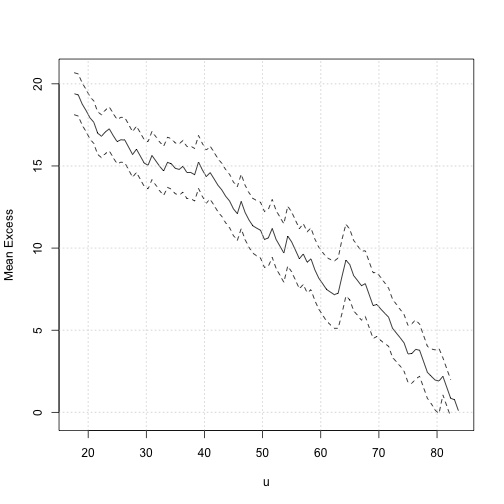The 95% confidence band is shown in gray. There is a general decrease of the mean excess with increasing intensity levels. The decrease is linear above a value of about 62 m s$$^{-1}$$ indicating that any threshold chosen above this intensity results in a set of wind speeds that follow a GPD.

To maximize the number of wind speed values for estimating the model parameters, the lowest such threshold is the optimal choice.

Alternatively you can proceed by trial-and-error. You calculate the parameters of the GPD for increasing thresholds and choose the minimum threshold at which the parameter values remain nearly constant.

## Categorical Wind Speeds by County

Hurricane wind speeds are often given by Saffir-Simpson category. While this is can be useful for presentations, you should avoid using categories for analysis and modeling. However, historical data are sometimes provided only by category.

Here you model county-level categorical wind data. The data represent direct and indirect hurricane hits by Saffir-Simpson category and were described and organized in Chapter 6.

The wind speed category and count data are saved in separate binary files. Make them available in your working directory by typing

load("catwinds.RData")


The list of data frames is stored in the object winds. Recall that lists are generic objects and can be of any type. To see the data frame for Cameron County, Texas (the first county in the list where the counties are numbered from 1 to 175 starting with south Texas), type

winds[]

##   Year W.time1 W.time2 W.status
## 1 1909      42      50        3
## 2 1909      33      50        3
## 3 1910      33      42        3
## 4 1919      33      50        3
## 5 1933      42      50        3
## 6 1933      50      58        3
## 7 1967      50      58        3
## 8 1980      50      58        3
## 9 2008      33      42        3


The data frame contains a numerical year variable and a categorical survival variable. The survival variable has three components with the first two indicating the wind speed bounds of the cyclone. The bounds correspond to Saffir-Simpson cyclone categories.

The data frame of corresponding hurricane counts is stored in the object counts. To see the first ten years of counts from Cameron County, type

counts[1:10, 1]

## 1900 1901 1902 1903 1904 1905 1906 1907 1908 1909
##    0    0    0    0    0    0    0    0    0    2


There were no hurricanes in this part of Texas during the first nine years of the 20th century, but there were two in 1909. The first eight county names are printed by typing

colnames(counts)[1:8]

##  "CAMERON"      "WILLACY"      "KENEDY"       "KLEBERG"
##  "NUECES"       "SAN_PATRICIO" "ARANSAS"      "REFUGIO"


You use the two-parameter Weibull distribution to model the wind speed category data. The survival function ($$S(w)=P(W>w)$$) for the Weibull distribution ($$W \sim \mathrm{Weib}(b,a)$$) is $S(w)=\exp (- \left(\frac{w}{b}\right)^a)$ where $$a$$ and $$b$$ are the shape and scale parameters, respectively.

The Weibull distribution has the nice property that if $$W \sim \mathrm{Weib}(a,b)$$, then a linear transformation of $$W$$ results in a variable whose distribution is also Weibull [i.e. $$kW \sim \mathrm{Weib}(kb,a)$$].

Similarly, a power transformation results in a variable whose distribution is Weibull [i.e. $$W^k \sim \mathrm{Weib}(b^k,a/k)$$].

The county-level data has a lower and upper wind speed bound. This is called censored data. You know the wind speed is at least as strong as the lower bound but as strong or weaker than the upper bound.

Censored data attaches time1 and time2 to the bounds, but here the winds are from the same time. In other words, $$W$$ lies in an interval $$[W_l, W_u]$$ and the true wind speed follows a Weibull distribution.

So instead of using the logarithm of the density function in the Weibull likelihood, you use the logarithm of the probability distribution function over the interval.

### Marked Poisson process

These data were originally modeled in Jagger et al. (2001). The model considers annual winds by keeping only the highest wind event for that year. That is, a county that was hit by multiple hurricanes in a given year, only the strongest wind was used.

Here you reframe the analysis using a marked Poisson process meaning the wind events are independent and the number of events follows a Poisson distribution with a rate $$\lambda$$. The marks are the wind speed interval associated with the event. In this way, all events are included.

You assume the marks have a Weibull distribution with shape parameter $$a$$ and scale parameter $$b$$. The scale parameter has units of wind speed in m s$$^{-1}$$. Note that the mean exceedance wind speed is given by $$\mu=b\Gamma(1+1/a)$$ as can be seen by integrating the survival function.

The probability that the yearly maximum wind is less than or equal to $$w$$ can be found by determining the probability of not seeing a wind event of this magnitude.

Given the rate of events ($$\lambda$$) and the probability of an event exceeding $$w$$, the rate of events exceeding $$w$$ is a thinned Poisson process with rate given by $r(w)=\lambda \exp\left(-(w/b)^a\right)$

So the probability of observing no events is $$\exp(-r(w))$$ and thus the probability distribution of the yearly maximum winds is given by $F_{max}(w) = \exp\left(-\lambda \exp(-(w/b)^a)\right).$

The return level ($$w$$) in years ($$n$$) associated with the return period is given $$1/n=1-F(w)$$, the long run proportion of years with events exceeding $$w$$. Solving for $$w$$ gives $w = b \left(\log \left(\frac{\lambda}{\log \left(\frac{n}{n-1}\right)} \right)\right)^{\frac{1}{a}}$ which is approximately $w \approx b \left(\log (\lambda (n-.5) )\right) ^ \frac{1}{a}.$

### Return levels

To help with the modeling we packaged the functions Weibull survival [sWeib()], distribution of maximum winds [sWeibMax()], and return level [rlWeibPois()] in the file CountyWinds.R. Use the source() function to input these functions by typing

source("CountyWinds.R")

## Loading required package: MASS


To see how these functions work, suppose the annual hurricane rate for a county is $$\lambda$$ = 0.2 and the Weibull survival parameters are $$a$$ = 5 and $$b$$ = 50~m s$$^{-1}$$. Then, to estimate the return level associated with a 100-year return period hurricane wind event, you type

rlWeibPois(n = 100, a = 5, b = 50, lambda = 0.2)

##        100
## [1,] 62.25


Thus you can expect to see a hurricane wind of intensity at least 62.2 m s$$^{-1}$$ in the county once every 100 years on average.

Note that since the event frequency is 1 in 5 years (0.2), the return period in years is given by 1/[1-exp(-0.2)] or

round(1/(1 - exp(-0.2)))

##  6


The Weibull distribution has support on the real number line to positive infinity. This means there will be a non-zero probability of a wind exceeding any magnitude. Physically this is unrealistic.

You can generate a series of return levels using the rlWeibPois() function and the assigned parameters by typing

rl = round(rlWeibPois(n = c(5, 10, 20) * 10^(rep(0:2, each = 3)), a = 5, b = 50,
lambda = 0.2), 1)
rl

##        5   10   20 50  100  200  500 1000 2000
## [1,] NaN 45.7 53.2 59 62.2 64.9 67.9 69.8 71.5


On average, the county can expect to see a cyclone of 45.7 m s$$^{-1}$$ once every 10 years. For a given return period, the return level scales linearly with the scale parameter $$b$$, but to a power of $$1/a$$ with the shape parameter. Note that the function returns an NaN (not a number) for the 5-year return level since it is below 33 m s$$^{-1}$$.

### Covariates

The return level computation above assumes all years have equal probability of events and equal probability of wind speed exceedances. This is a climatology model.

You might be able to do better by conditioning on environmental factors. You include covariate affects by modeling the transformed parameters $$\log \lambda$$, $$\log b$$ and $$\log a$$ as linear functions of the covariates NAO, SST, SOI and SSN.

For a given county, let $$L_i$$ and $$U_i$$ be the lower and upper bounds for each observation and $$y_j$$ be the yearly cyclone count. Further, assume that $$[\theta_{\lambda},\theta_b,\theta_a]$$ is a vector of model parameters associated with covariate matrices given as $$X_{\lambda}$$,$$X_{b}$$ and $$X_{a}$$ of size $$m \times p_{\lambda}$$,$$n \times p_a$$ and $$n \times p_b$$, respectively.

The log likelihood function of the process for a given county with $$n$$ observations over $$m$$ years is $\mathrm{LL}(\theta) = \sum_{i=1}^n \log\left(\exp(-(L_i/b_i)^{a_i}) -\exp(-(U_i/b_i)^{a_i})\right) + \\ \sum_{j=1}^m y_j \log(\lambda_i) -\lambda_i - \log(i!)\\ \log(a_i) = X_{a}[i,]\cdot \theta_a \\ \log(b_i) = X_{mu}[i,] \cdot \theta_{b} \\ \log(\lambda_i) = X_{\lambda}[i,] \cdot \theta_{\lambda} \\$ The log likelihood separates into two parts one for the counts and another for the wind speeds. This allows you to use maximum likelihood estimation (MLE) of the count model parameters separately from the wind speed model parameters. The count model is a generalized linear model and you can use the glm() function.

For the wind speeds, you can build the likelihood function (see Jagger et al. 2001) or using a package. The advantage of the latter is greater functionality through the use of plot, summary, and predict methods.

You can usually find a package to do what you need using familiar methods. If not, you can write an extension to an existing package. If you write an extension send it to the package maintainer so your functionality gets added to future versions.

The gamlss package together with the gamlss.dist package provide extensions to the glm() function from the stats package and to the gam() function from the gam package for generalized additive models.

The gamlss.cens package allows you to fit parametric distributions to censored and interval data created using the Surv() function in the Survival package for use with the gamlss.dist package. With this flexibility you can estimate the parameters of the return level model without the need to writing code for the likelihood or its derivatives.

You make the packages available to your working directory by typing

require(gamlss)
require(gamlss.cens)


You are interested in estimating return levels at various return periods. You can do this using the rlWeibPois() function as described above. The estimates have a degree of uncertainty due to finite sample size.

The estimated model parameters also have uncertainty. You propagate this uncertainty to your final return level estimates in at least two ways. One is to estimate the variance of the return level as a function of the parameter covariance matrix (delta method).

Another is to sample the parameters assuming they have a normal distribution with a mean equal to the MLE estimate and with a variance-covariance matrix given by $$\Sigma$$, where $$\Sigma$$ is a block diagonal matrix composed of a $$p_\lambda \times p_\lambda$$ covariance matrix from the count model and a $$p_a + p_b \times p_a + p_b$$ covariance matrix from the wind speed model.

The parameters and the covariances are returned from the vcov() function on the model object returned from glm() and gamlss().

First you generate samples of the transformed parameters and save them in separate vectors ($$\log \lambda, \log b, \log a$$). Then you take the antilog of the inner product of the parameters and the corresponding set of their predictions based on the covariates.

You then pass these values and your desired return periods to rlWeibPois() to obtain one return level for each return period of interest. Finally you use the function sampleParameters() provided in CountyWinds.R to sample the return levels for a given set of predictors and return periods.

Here you model the categorical wind data for Florida's Miami-Dade County. The model can be applied to any county that has experienced more than a few hurricanes. Since not all counties have the same size, comparing wind probabilities across counties is not straightforward. On the other hand, county-wide return levels are useful to local officials. You model the county data with and without the covariates.

First you extract the wind speed categories and the counts.

miami.w = winds[]
Year = as.numeric(row.names(counts))
H = counts[, 57]
miami.c = data.frame(Year = Year, H = H)


Since you have two separate data sets, it is a good idea to see if the cyclone counts match the winds by year and number. You do this by typing

all(do.call("data.frame", rle(miami.w$Year)) - miami.c[miami.c$H > 0, c(2, 1)] ==
0)

##  TRUE


You first fit the counts to a Poisson distribution by typing

fitc = glm(H ~ 1, data = miami.c, family = "poisson")


Next you fit the wind speed intervals to a Weibull distribution by typing

WEIic = cens(WEI, type = "interval", local = FALSE)
fitw = gamlss(W ~ 1, data = miami.w, family = WEIic, trace = FALSE)
rm(list = c("W", "Year"))  #Bug


Finally you generate samples of return levels for a set of return periods by typing

rp = c(5, 10, 20) * 10^(rep(0:2, each = 3))
rl = sampleParameters(R = 1000, fitc = fitc, fitw = fitw, n = rp)


You display the results with a series of box plots. You do this for Miami-Dade, FL and Galveston, TX.

par(mfrow = c(1, 2), las = 1, mgp = c(2, 0.4, 0), tcl = -0.3)
xvalues = 1:dim(rl)
plot(rp, rep(50, dim(rl)), type = "n", log = "x", ylim = c(20, 80), xlab = "Return period [yr]",
ylab = "Return level [m/s]")
grid(equilogs = FALSE)
mtext("a", side = 3, line = 1, adj = 0, cex = 1.1)
for (i in xvalues) {
points(rp[i], quantile(rl[, 1, i], prob = 0.5, na.rm = TRUE), pch = 19,
col = "black")
lines(c(rp[i], rp[i]), c(quantile(rl[, 1, i], prob = 0.25, na.rm = TRUE),
quantile(rl[, 1, i], prob = 0.025, na.rm = TRUE)))
lines(c(rp[i], rp[i]), c(quantile(rl[, 1, i], prob = 0.75, na.rm = TRUE),
quantile(rl[, 1, i], prob = 0.975, na.rm = TRUE)))
}
galv.w = winds[]
Year = as.numeric(row.names(counts))
H.g = counts[, 13]
galv.c = data.frame(Year = Year, H.g = H.g)
fitc.g = glm(H.g ~ 1, data = galv.c, family = "poisson")
WEIic.g = cens(WEI, type = "interval", local = FALSE)
fitw.g = gamlss(W ~ 1, data = galv.w, family = WEIic.g, trace = FALSE)
rm(list = c("Year"))
rp.g = c(5, 10, 20) * 10^(rep(0:2, each = 3))
rl.g = sampleParameters(R = 1000, fitc = fitc.g, fitw = fitw.g, n = rp.g)
plot(rp.g, rep(50, dim(rl.g)), type = "n", log = "x", ylim = c(20, 80), xlab = "Return period [yr]",
ylab = "Return level [m/s]")
grid(equilogs = FALSE)
mtext("b", side = 3, line = 1, adj = 0, cex = 1.1)
for (i in xvalues) {
points(rp.g[i], quantile(rl.g[, 1, i], prob = 0.5, na.rm = TRUE), pch = 19,
col = "black")
lines(c(rp.g[i], rp.g[i]), c(quantile(rl.g[, 1, i], prob = 0.25, na.rm = TRUE),
quantile(rl.g[, 1, i], prob = 0.025, na.rm = TRUE)))
lines(c(rp.g[i], rp.g[i]), c(quantile(rl.g[, 1, i], prob = 0.75, na.rm = TRUE),
quantile(rl.g[, 1, i], prob = 0.975, na.rm = TRUE)))
}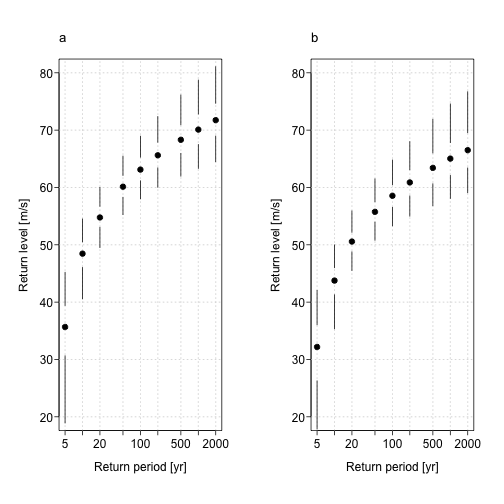The median return level is shown with dot. Given the model and the data a 50-year return period is a hurricane that produces winds of at least 60 m s$$^{-1}$$ in the county. The return level increases with increasing return period. The uncertainty levels represent the upper and lower quartile values and the ends of the whiskers define the 95% confidence interval.

Andrew struck Miami in 1992 as a category 5 hurricane with 70 m s$$^{-1}$$ winds. Your model indicates that the most likely return period for a cyclone of this magnitude is 1000 years, but it could be as short as 100 years. Return levels are higher at all return periods for Miami compared to Galveston. Miami is closer to the main tropical cyclone development region of the North Atlantic.

A categorical wind speed model can be used on tornado data where intensities are estimated in intervals defined by the Fujita scale.